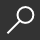## Greek Mathematical Works, Volume I : Thales to Euclid by Ivor Thomas

Overview -

The wonderful achievement of Greek mathematics is here illustrated in two volumes of selected mathematical works. Volume I contains: The divisions of mathematics; mathematics in Greek education; calculation; arithmetical notation and operations, including square root and cube root; Pythagorean arithmetic, including properties of numbers; square root of 2; proportion and means; algebraic equations; Proclus; Thales; Pythagorean geometry; Democritus; Hippocrates of Chios; duplicating the cube and squaring the circle; trisecting angles; Theaetetus; Plato; Eudoxus of Cnidus (pyramid, cone, etc.); Aristotle (the infinite, the lever); Euclid.

Volume II (Loeb Classical Library no. 362) contains: Aristarchus (distances of sun and moon); Archimedes (cylinder, sphere, cubic equations; conoids; spheroids; spiral; expression of large numbers; mechanics; hydrostatics); Eratosthenes (measurement of the earth); Apollonius (conic sections and other works); later development of geometry; trigonometry (including Ptolemy's table of sines); mensuration: Heron of Alexandria; algebra: Diophantus (determinate and indeterminate equations); the revival of geometry: Pappus.

local_shippingFor DeliveryLimited Availability. Ships in 2-4 weeks.
This item is Non-Returnable.
FREE Shipping for Club Members helpNew & Used Marketplace 7 copies from \$30.45

## More About Greek Mathematical Works, Volume I by Ivor Thomas

### Overview

The wonderful achievement of Greek mathematics is here illustrated in two volumes of selected mathematical works. Volume I contains: The divisions of mathematics; mathematics in Greek education; calculation; arithmetical notation and operations, including square root and cube root; Pythagorean arithmetic, including properties of numbers; square root of 2; proportion and means; algebraic equations; Proclus; Thales; Pythagorean geometry; Democritus; Hippocrates of Chios; duplicating the cube and squaring the circle; trisecting angles; Theaetetus; Plato; Eudoxus of Cnidus (pyramid, cone, etc.); Aristotle (the infinite, the lever); Euclid.

Volume II (Loeb Classical Library no. 362) contains: Aristarchus (distances of sun and moon); Archimedes (cylinder, sphere, cubic equations; conoids; spheroids; spiral; expression of large numbers; mechanics; hydrostatics); Eratosthenes (measurement of the earth); Apollonius (conic sections and other works); later development of geometry; trigonometry (including Ptolemy's table of sines); mensuration: Heron of Alexandria; algebra: Diophantus (determinate and indeterminate equations); the revival of geometry: Pappus.

This item is Non-Returnable.

### Details

• ISBN-13: 9780674993693
• ISBN-10: 0674993691
• Publisher: Harvard University Press
• Publish Date: January 1939
• Page Count: 576
• Dimensions: 6.7 x 4.63 x 1.08 inches
• Shipping Weight: 0.8 pounds

Series: Loeb Classical Library #335Related Categories